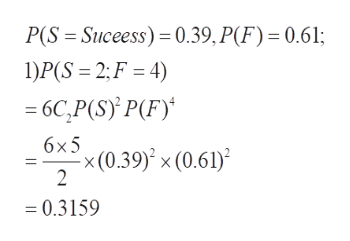# A Bernoulli process has 6 trials and probability of success 0.39. Find the following probabilities.1) Exactly 2 successes.2) Exactly 2 failures3) a) At most 1 success. b) At least 1 success. c) At least 1 success and 1 failure.

Question
128 views

A Bernoulli process has 6 trials and probability of success 0.39. Find the following probabilities.

1) Exactly 2 successes.

2) Exactly 2 failures

3) a) At most 1 success. b) At least 1 success. c) At least 1 success and 1 failure.
check_circle

Step 1

As per norms , the first three questions are answered. To determine the required probabilities in a Bernoulli process with the given parameters

Step 2

Given an experiment  (trial) with P(success) =0.39; so P(failure) = 0.61. This trial is repeated 6 times, and we need to calculate the required probabilities

Step 3

1) Exactly 2 successes if and only if exactly 4 failures. Any 2 of the 6 trials could be ...help_outlineImage TranscriptioncloseP(S Suceess) 0.39, P(F) = 0.61; 1)P(S 2; F 4) - 6C,P(S) P(F) 6xx(0.39) x (0.61) 2 = 0.3159 fullscreen

### Want to see the full answer?

See Solution

#### Want to see this answer and more?

Solutions are written by subject experts who are available 24/7. Questions are typically answered within 1 hour.*

See Solution
*Response times may vary by subject and question.
Tagged in

### Probability